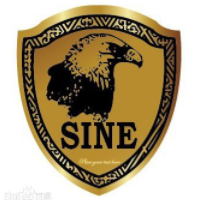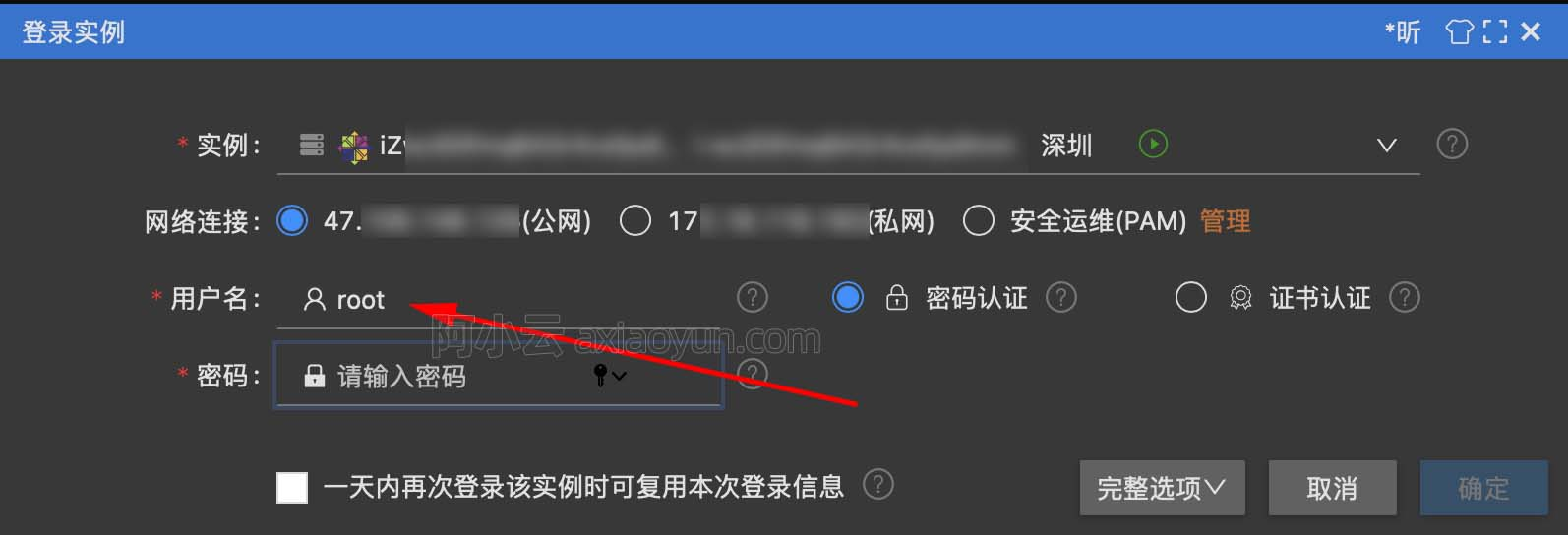BZOJ 1083: [SCOI2005]繁忙的都市【Kruscal最小生成树裸题】

+关注继续查看

1083: [SCOI2005]繁忙的都市

Time Limit: 10 Sec  Memory Limit: 162 MB
Submit: 2925  Solved: 1927
[Submit][Status][Discuss]

Description

城市C是一个非常繁忙的大都市，城市中的道路十分的拥挤，于是市长决定对其中的道路进行改造。城市C的道

Input

第一行有两个整数n,m表示城市有n个交叉路口，m条道路。接下来m行是对每条道路的描述，u, v, c表示交叉

Output

两个整数s, max，表示你选出了几条道路，分值最大的那条道路的分值是多少。

4 5
1 2 3
1 4 5
2 4 7
2 3 6
3 4 8

3 6

Source

In the mathematical field of graph theory, a spanning tree T of an undirected graph G is a subgraph that is a tree which includes all of the vertices of G. In general, a graph may have several spanning trees, but a graph that is not connected will not contain a spanning tree (but see Spanning forests below). If all of the edges of G are also edges of a spanning tree T of G, then G is a tree and is identical to T (that is, a tree has a unique spanning tree and it is itself).Paste_Image.png

kruscal算法的模板代码如下：

1 const int maxn=400;//最大点数
2 const int maxm=10000;//最大边数
3 int n,m;//n表示点数，m表示边数
4 struct edge{int u,v,w;} e[maxm];//u,v,w分别表示该边的两个顶点和权值
5 bool cmp(edge a,edge b)
6 {
7     return a.w<b.w;
8 }
9 int fa[maxn];//因为需要用到并查集来判断两个顶点是否属于同一个连通块
10 int find(int x)
11 {
12     if(x==fa[x]) return x;
13     else return fa[x]=find(fa[x]);
14 }
15 int kruscal()
16 {
17     int ans=-1;
18     sort(e+1,e+1+m,cmp);
19     for(int i=1;i<=n;++i) fa[i]=i;//初始化并查集
20     int cnt=n;
21     for(int i=1;i<=m;++i)
22     {
23         int t1=find(e[i].u);
24         int t2=find(e[i].v);
25         if(t1!=t2)
26         {
27             if(cnt==1) break;
28             fa[t1]=t2;
29             ans=max(ans,e[i].w);
30             cnt--;
31         }
32     }
33     return ans;
34 }

1 #include <bits/stdc++.h>
2 using namespace std;
3 const int maxn=400;///最大点数
4 const int maxm=10000;///最大边数
5 int n,m;///n表示点数,m表示边数
6 struct edge
7 {
8     int u,v,w;///u,v,w分别表示该边的两个顶点和权值
9 }e[maxm];
10 bool cmp(edge a,edge b)
11 {
12     return a.w<b.w;
13 }
14 int fa[maxn];///判断两个点是否属于同一个连通块
15 int find(int x)
16 {
17     if(x==fa[x])
18         return x;
19     else return fa[x]=find(fa[x]);
20 }
21 int kruscal()
22 {
23     int ans=-1;
24     sort(e+1,e+1+m,cmp);
25     for(int i=1;i<=n;i++)
26         fa[i]=i;///初始化并查集
27     int cnt=n;
28     for(int i=1;i<=m;i++)
29     {
30         int t1=find(e[i].u);
31         int t2=find(e[i].v);
32         if(t1!=t2)
33         {
34             if(cnt==1)
35                 break;
36             fa[t1]=t2;
37             ///ans+=e[i].w;
38             ans=max(ans,e[i].w);
39             cnt--;
40         }
41     }
42     return ans;
43 }
44 int main()
45 {
46     cin>>n>>m;
47     for(int i=1;i<=m;i++)
48     {
49         cin>>e[i].u>>e[i].v>>e[i].w;
50     }
51     cout<<n-1<<" ";
52     cout<<kruscal()<<endl;
53     return 0;
54 }9494 06334 014376 012036 013177 0windows server 2008阿里云ECS服务器安全设置

9052 04620 06791 054290 04012 0
angel_kitty

707

1

《2021云上架构与运维峰会演讲合集》

《零基础CSS入门教程》

《零基础HTML入门教程》